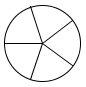### Home > CC1MN > Chapter 8 > Lesson 8.2.1 > Problem8-77

8-77.

Include a sketch and a division number sentence to support each of your answers below.

1. How many fifths are there in a whole?2. How many thirds are there in $2\frac{1}{3}$?

$\text{There are } \frac{3}{3}\text{ in 1.}$ Use this information to help you.

3. How many $\frac { 2 } { 5 }$ are there in $4$?

Use the picture below to help with this one.